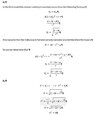# Consider three models of the Mass distribution in the Milky Way

Barbequeman
Homework Statement:
Consider three models of the mass distribution in the Milky Way. Model 1 has a constant circular velocity Vc. Model 2 places all the mass of the Milky Way at a single point at the centre of the Galaxy. Model 3 consists of a system in which the mass of the system is distributed in a sphere of constant density. In this model you can use Newton’s Theorem to compute the circular velocity.
The following rule about derivatives can be used. Suppose you have a function:

Question A. is
For each of the three models, write down the equation of the circular velocity as a function of radius. (Hints: For model 1 this should be trivial. For model 2 equate the gravitational and centrifugal forces. For model 3 follow a similar procedure to what you did for model 2, but considering the mass enclosed at a given radius.)

Question B. is
Therefore, for each of the three models, calculate dV/dR
Relevant Equations:
V=V_0*R^n
then the derivative is given by:
dV/dR=V_0nR^n-1
I attached a Jpeg with my attempted solution but I´m not sure if I´m on the right way... I hope for a correction in my calculations

#### Attachments

•Aufgabe2_solutionattempt.jpg
18.4 KB · Views: 29

You did not delineate where you are working on case 1, 2 or 3. It's hard to see what the intent of the calculations was.

In model 1 you are given the relationship a priori. You know what V(r) is. There's nothing to calculate. You are told the velocity is constant at all radii. That's why the question states it's trivial. For model 1 you should have stopped at the first line.

In line two you compare the centripetal force with the gravitational force, as you are required for both 2) and 3). But the equation for the centripetal force is incorrect. Check your notes.

At no point should you be assuming a flat-disc distribution of matter. You are given two cases (apart from the trivial one, where the distribution of matter is of no concern): case 2) all mass is at the point in the centre, and case 3) all mass is in spherical distribution. Case 3) requires you to think about how the effective gravitational mass of the Galaxy M changes with radius. Recall what Newton's shell theorem says about mass enclosed in a sphere and mass above the sphere.

•Barbequeman
Homework Helper
Gold Member
2022 Award
In case 2, what is rotating?

This is not the correct approach.
You've introduced some extra masses here, that I can't see the reason for. You will only really have one mass to deal with here - the mass attracting the point particle to the centre of the galaxy. The mass of the orbiting particle will cancel out when you compare the gravitational force with the centripetal force.

Note, you were on the right track with the first four lines in your first attempt at solution in the opening post - with the exception of incorrectly stating the equation for the centripetal force.
Take heart, the whole exercise involves relatively simple calculations, once you see what's going on.

But let's take it one step at a time. Question a) for model 1.
Can you first state an example of a function f(x) that is constant for all x?

Don't jump to subsequent points until we've sorted this one out first.

(FYI the forum supports LaTeX, if that's something you're comfortable writing equations in)